Nuclear energy E = mc2 The nucleus of an atom contains protons and neutrons. Now we know that like charges repel, so why do we find positive charges(protons) packed so close together in the nucleus? The presence of a very strong force called a nuclear force, that exists between the nucleons (protons and neutrons) within the nucleus, is the reason. This force is up to 1,000 times stronger than electrostatic force repelling the protons apart.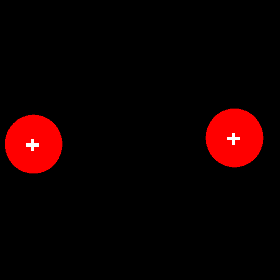Electrostatic forces repel like charges and prevent them from getting close to each other. These two protons do not have the kinetic energy required to overcome the electrostatic repulsion.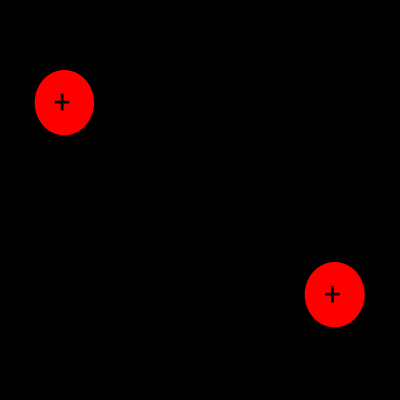At temperatures around 10 million degrees Celsius nuclear fusion takes place. These temperatures can be achieved in the core of stars such as our own sun and during themonuclear explosions. The nuclear force only operates at distances less than 10-15 metres. At distances greater than 10-15 metres it is absent. Electrostatic forces on the other hand operate over longer distances. So in order for two protons to come close enough so that the nuclear force takes hold they must first overcome the electrostatic forces. Protons overcome the electrostatic forces at temperatures in excess of 107 oC. Only at such high temperatures do the protons have enough energy to overcome the force of repulsion and get within 10-15 metres of each other where the nuclear force can fuse them together. When nucleons (protons and neutrons) combine to form a nucleus the total mass is slightly less than the sum of all the nucleons. How can this happen? How can mass be lost? What happened to the "Law of Conservation of Mass?" Mass is converted into energy(kinetic and heat) according to the equation below. E = mc2 This implies that mass is a form of potential energy that can be released during nuclear reactions. This is exactly what happens. Take the formation of neon for example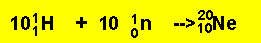10 protons (each with relative mass of 1.007825) and 10 neutrons(each with relative mass of 1.00865) combine to form a neon nucleus with a mass of 19.9924. The mathematics is simple 10 X 1.007825 + 10 X 1.00865 = 20.1648 but the nucleus has a mass of 19.9924 there is a shortfall of 0.1724 mass units. This mass was released as energy during the fusion reaction. The mass loss that occurs when nucleons fuse, is different for the formation of different nuclei. The formation of helium from the fusion of 4 hydrogen nuclei(protons) can be written in a nuclear equation such as the one below.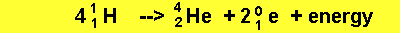As we can see, energy is given off, called the nuclear binding energy. The more energy per nucleon that is given off the more stable the nucleus. In other words the more energy we have to input in order to break the nucleus into its constituent nucleons according to the equation below.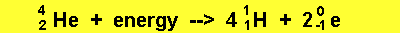Exercises Nuclear equations Continue

Home